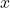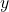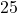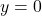Question

The equation below represents the amount of money left in Brianna’s pocket after buying donuts for her friends:
y = – 1.25x + 25
a)Explain what each value in the equation represents.
b)What is the greatest number of donuts Brianna can buy

1.Part (a)
Given equation:•= number of donuts Brianna buys
•= total amount of money in Brianna’s pocket (in dollars)
•= For every donut Brianna buys, the money in her pocket decreases by $1.25 ⇒ each donut costs$.125
•= Total amount of money Brianna has before she buys any donuts (in dollars) ⇒ \$25.00
Part (b)
The greatest number of donuts Brianna can buy is when the money she has is reduced to zero dollars.  Therefore, substituteinto the equation and solve for:Therefore, the greatest number of donuts Brianna can buy is 20 donuts.

2.